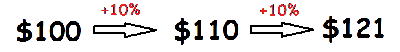Math Calculators, Lessons and Formulas

It is time to solve your math problem

mathportal.org

# Compound interest calculator

Compound Interest is calculated on the initial payment and also on the interest of previous periods.

Example: Suppose you give \$100 to a bank which pays you 10% compound interest at the end of every year. After one year you will have \$100 + 10% = \$110, and after two years you will have \$110 + 10% = \$121.Problem How much money would you need to deposit today at 12% annual interest compounded quarterly to have$1000 in the account after 2 years?

Result:

The principal is 789.41. Explanation To find amount we use formula:  $$A = P \left( 1 + \frac{r}{n} \right)^{\Large{n \cdot t}}$$ A = total amount P = principal or amount of money deposited, r = annual interest rate n = number of times compounded per year t = time in years In this example we have $$A = \1000 ~,~ r = 12 \% ~ , ~ n = 4 ~ \text{and} ~ t = 2 ~ \text{years}$$ After plugging the given information we have \begin{aligned} 1000 &= P \left( 1 + \frac{ 0.12 }{ 4 } \right)^{\Large{ 4 \cdot 2 }} \\ 1000 &= P \cdot { 1.03 } ^ { 8 } \\ 1000 &= P \cdot 1.26677 \\ P &= \frac{ 1000 }{ 1.26677} \\ P &= 789.41 \end{aligned} ## Report an Error ! Script name : compound-interest-calculator Imputed values: 1 , 1 , 12 , 2 , 1 , 3 , 1000 Comment (optional) Email (optional) ## Share Result Share this result with others by using the link below. You can click here to verify link or you can just copy and paste link wherever you need it. Compound Interest Calculator 0 1 2 3 4 5 6 7 8 9 . del Show me the solution without an explanation Compound Interest Calculator 0 1 2 3 4 5 6 7 8 9 . del Show me the solution without an explanation examples example 1: What will a deposit of\color{blue}{\$4500}$ at $\color{blue}{7\%}$ compounded $\color{blue}{\text{yearly}}$ interest be worth if left in the bank for $\color{blue}{\text{9 years}}$ ?
example 2:
What will a deposit of $\color{blue}{\$3500}$at$\color{blue}{10\,\%}$compounded$\color{blue}{\text{monthly}}$be worth if left in the bank for$\color{blue}{8 \, \text{years}}$? example 3: How much money would you need to deposit today at$\color{blue}{8\% \, \text{annual}}$interest compounded$\color{blue}{\text{monthly}}$to have$\color{blue}{\$1200}$ in the account after $\color{blue}{12 \, \text{years}} \, \text{?}$
example 4:
Find the present value of $\color{blue}{\$1000}$to be received at the end of$\color{blue}{2 \, \text{years}}$at a$\color{blue}{12\%}$nominal annual interest rate compounded$\color{blue}{\text{quarterly}}$. example 5: What annual interest rate is implied if you lend someone$\color{blue}{\$1700}$ and are repaid $\color{blue}{\$ 1910}$in$\color{blue}{\text{two years}}$? example 6: Suppose that a savings account is compounded$\color{blue}{\text{monthly}}$with a principal of$\color{blue}{\$1350}$. After $\color{blue}{8 \, \text{months}}$, the amount increased to $\color{blue}{\$ 1424}$. What was the per annum interest rate? example 7: How long does it take for$\color{blue}{\$4300}$ to grow into $\color{blue}{\$6720}$at$\color{blue}{9\,\%}$compounded$\color{blue}{\text{quarterly}}\$?

Quick Calculator Search